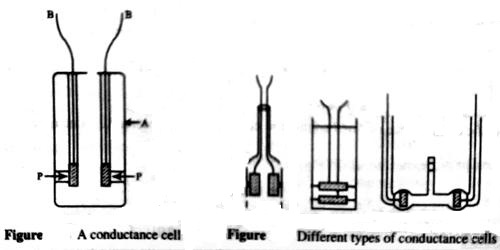# Conductance Cell: Cell Constant

Conductance Cell: Cell Constant

A conductance cell is a devise to measure the conductance of electrolytic solutions. Different forms of cells are in use for conductance measurement. A cell which is commonly used is shown in Figure 1. The container is a glass vessel, A, which carries two thick Platonized platinum (fine particles of platinum, black in color, deposited on the platinum foil electrolytically) foils, PP, securely fixed so that their distances are not altered. Two metallic wires, BB, sealed to the platinum foils and protected by glass tubes serve as the leads for connecting to the measuring bridge. Several other forms of cells are shown in Figure 2.It is known from equation (Conductance of Electrolytic Solutions) that the resistance of a conductor is given by

R = ρ x (l//a)

In the case of conductance cell ‘l’ is the distance between the two electrodes and a is the area of cross-section of the electrodes, as the resistance of the column of solution between the two electrodes is measured. For a particular cell (l/a), known as cell constant, is a constant. As the dimension of the electrodes and the distance between them can hardly be measured accurately the usual practice is to determine the cell constant by measuring the conductance of a solution whose specific conductance is accurately known at a particular temperature. Standard solutions of KCl are used for the purpose. The specific conductance of any solution may then be obtained from the equation (Conductance of Electrolytic Solutions).

Specific conductance = Observed conductance x cell constant.